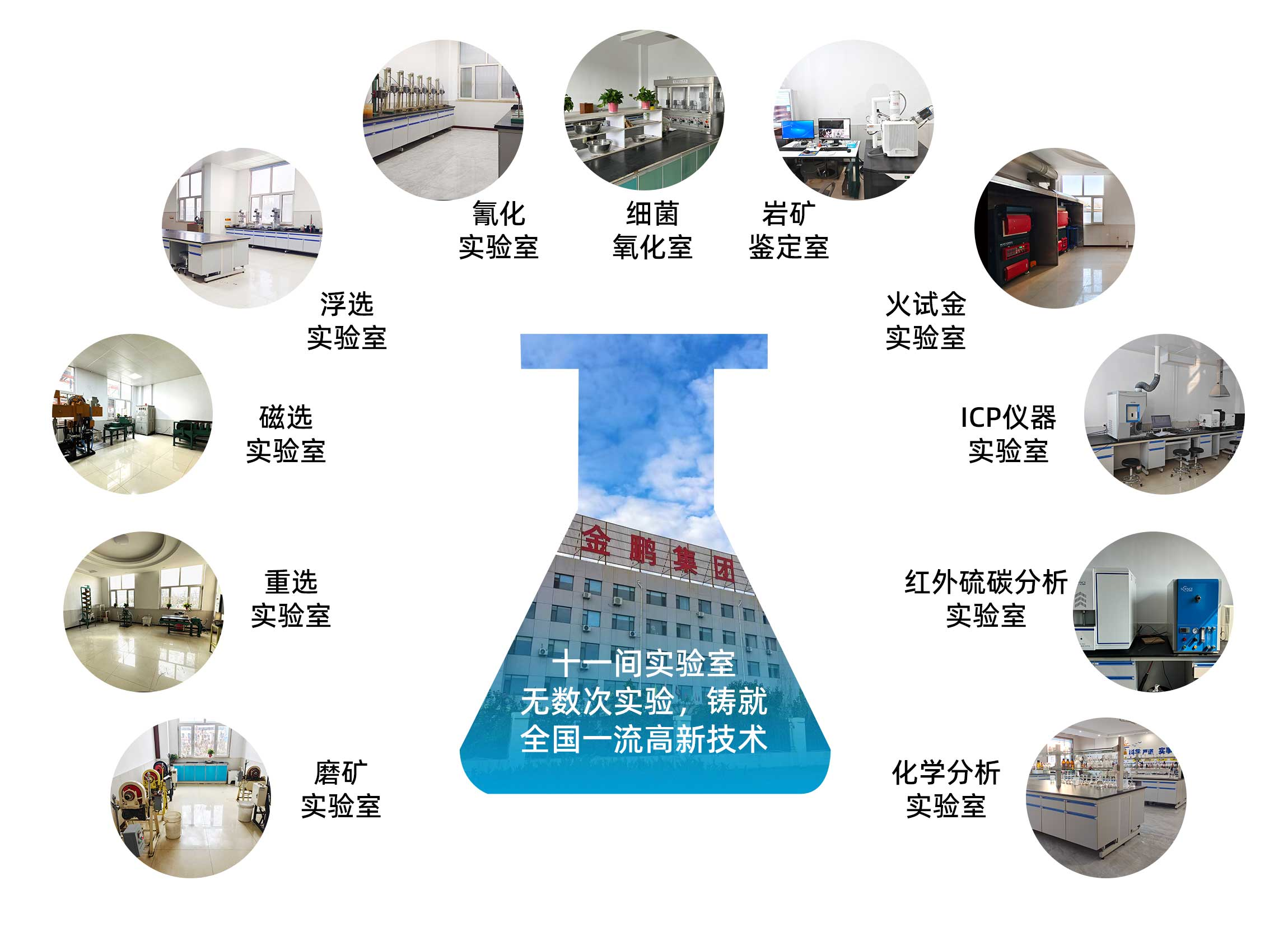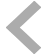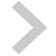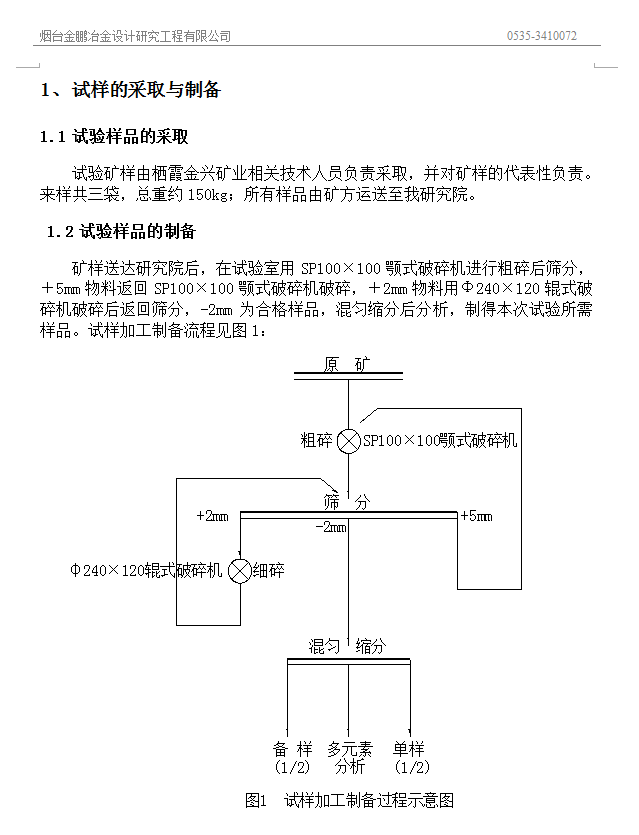实验室•褐铁矿呈细脉分布于裂隙中

•石英细脉薄片

•黄铁矿残留状分布褐铁矿中• c=20% 高度：48%
• c=25% 高度：57%
• c=30% 高度：76%
• c=40% 高度：94%
• c=20% 高度：38%
• c=25% 高度：51%
• c=30% 高度：55%
• c=40% 高度：90%
• c=20% 高度：36%
• c=25% 高度：50%
• c=30% 高度：52%
• c=40% 高度：88%
• c=20% 高度：32%
• c=25% 高度：42%
• c=30% 高度：49%
• c=40% 高度：85%
• c=20% 高度：29%
• c=25% 高度：42%
• c=30% 高度：47%
• c=40% 高度：80%————————————————————————————————

————————————————————————————————

•